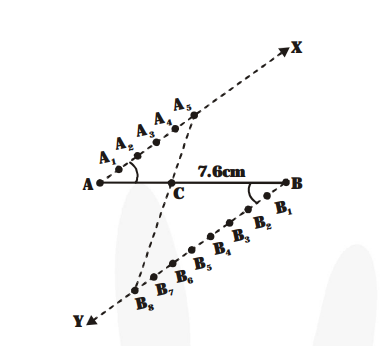# Draw a line segment of length 7.6 cm and divide it in the ratio 5 : 8.

Question:

Draw a line segment of length 7.6 cm and divide it in the ratio 5 : 8. Measure the two parts.

Solution:

Steps of construction :1. Draw AB = 7.6 cm.

2. AX is any ray making an acute angle with AB above the line AB.

3. Draw ray $B Y$ below the line $A B$ and parallel to the ray $A X$ by constructing $\angle A B Y=\angle B A X$.

4. Mark points $A_{1}, A_{2}, \ldots, A_{5}$ on $A X$ and $B_{1}, B_{2}$,

.....,$\mathrm{B}_{8}$ on $\mathrm{BY}$ such that $\mathrm{AA}_{1}=\mathrm{A}_{1} \mathrm{~A}_{2}=\ldots .=$

$A_{4} A_{5}=B B_{1}=B_{1} B_{2}=\ldots .=B_{7} B_{8} .$

5. Join $\mathrm{A}_{5} \mathrm{~B}_{\gamma}$. It intersects $\mathrm{AB}$ at $\mathrm{C}$.

Now, $\mathrm{C}$ divides $\mathrm{AB}$ in the ratio $5: 8$.